Courses

# RD Sharma Solutions Ex-24.1, (Part - 2), Measures Of Central Tendency, Class 9, Maths Class 9 Notes | EduRev

## Class 9 : RD Sharma Solutions Ex-24.1, (Part - 2), Measures Of Central Tendency, Class 9, Maths Class 9 Notes | EduRev

The document RD Sharma Solutions Ex-24.1, (Part - 2), Measures Of Central Tendency, Class 9, Maths Class 9 Notes | EduRev is a part of the Class 9 Course RD Sharma Solutions for Class 9 Mathematics.
All you need of Class 9 at this link: Class 9

Q 13. Explain,  by taking a suitable example, how the arithmetic mean alters by

(i) adding a constant k to each term,
(ii) Subtracting a constant k from each term,
(iii) multiplying each term by a constant k and
(iv) dividing each term by non-zero constant k.

SOLUTION :

Let say numbers are 3 , 4 , 5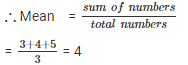(i). Adding constant term k = 2 in each term.

New numbers are = 5 , 6 , 7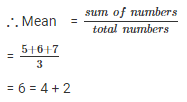∴ new  mean will be 2 more than the original mean.

(ii). Subtracting constant term k = 2 in each term.

New numbers are = 1 , 2 , 3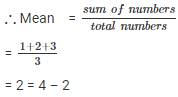∴ new  mean will be 2 less than the original mean.

(iii) . Multiplying by constant term k = 2 in each term.

New numbers are = 6 , 8 , 10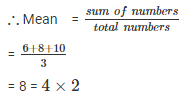∴ new  mean will be 2 times of  the original mean.

(iv) . Divide the constant term k =2 in each term.

New numbers are = 1.5 , 2 , 2.5.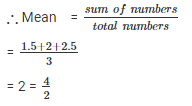∴ new  mean will be half  of  the original mean.

Q 14. The mean of marks scored by 100 students was found to be 40. Later on, it was discovered that a score of 53 was misread as 83. Find the correct mean.

SOLUTION :

Mean marks of 100 students = 40

Sum of marks of 100 students = 100×40

= 4000

Correct value = 53

Incorrect value = 83

Correct sum = 4000 – 83 + 53 = 3970

∴ correct  mean = 3970/100 = 39.7

Q 15 . The traffic police recorded the speed (in km/hr) of 10 motorists as 47 , 53 , 49 , 60 , 39 , 42 , 55 , 57 , 52 , 48 . Later on, an error in recording instrument was found. Find the correct average speed of the motorists if the instrument is recorded 5 km/hr less in each case.

SOLUTION :

The speed of 10 motorists are 47 , 53 , 49 , 60 , 39 , 42 , 55 , 57 , 52 , 48 .

Later on it was discovered that the instrument recorded 5 km/hr less than in each case

∴ correct values are = 52 , 58 , 54 , 65 , 44 , 47 , 60 , 62 , 57 , 53.

∴ correct  mean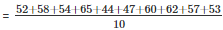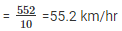Q 16. The mean of five numbers is 27. If one number is excluded, their mean is 25. Find the excluded number.

SOLUTION :

The mean of five numbers is 27

The sum of five numbers = 5×27 = 135

If one number is excluded , the new mean is 25

∴Sum of 4 numbers = 4×25 = 100

∴ Excluded number = 135 – 100 = 35

Q 17. The mean weight per student in a group of 7 students is 55 kg. The individual weights of 6 of them (in kg) are 52, 54, 55, 53, 56 and 54. Find the weight of the seventh student.

SOLUTION :

The mean weight per student in a group of 7 students = 55 kg

Weight of 6 students (in kg) =  52 , 54 , 55 , 53 , 56 and 54

Let the weight of seventh student = x kg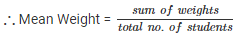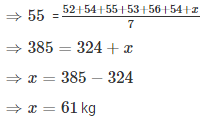∴ weight of seventh student = 61 kg.

Q 18. The mean weight of 8 numbers is 15. If each number is multiplied by 2 , what will be the new mean?

SOLUTION :

We have ,

The mean weight of 8 numbers is 15

Then , the sum of 8 numbers = 8×15 = 120

If each number is multiplied by 2

Then , new mean = 120×2 = 240

∴ new  mean = 240/8 = 30.

Q 19. The mean of 5 numbers is 18. If one number is excluded, their mean is 16. Find the excluded number.

SOLUTION :

The mean of 5 numbers is 18

Then , the sum of 5 numbers = 5×18 = 90

If one number is excluded

Then , the mean of 4 numbers = 16

∴ sum of 4 numbers = 4×16 = 64

Excluded number = 90 – 64 = 26.

Q 20. The mean of 200 items was 50. Later on, it was on discovered that the two items were misread as 92 and 8 instead of 192 and 88. Find the correct mean.

SOLUTION :

The mean of 200 items = 50

Then the sum of 200 items = 200×50 = 10,000

Correct values = 192 and 88.

Incorrect values = 92 and 8.

∴ correct sum = 10000 – 92 – 8 + 192 + 88 = 10180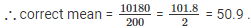Q 21 . Find the values of n and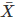in each of the following cases :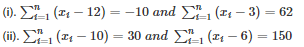SOLUTION :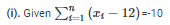⇒ (x1−12)+(x2−12)+ ……… +(xn−12) = −10

⇒(x1+x2+x3+x4+x5+⋅⋅⋅+xn)−(12+12+12+12+⋅⋅⋅⋅+12)=−10

⇒ ∑ x−12n =−10⋅⋅⋅⋅⋅⋅⋅⋅⋅⋅⋅⋅⋅⋅⋅(1)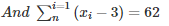⇒ (x1−3)+(x2−3)+⋅⋅⋅⋅⋅+(xn−3) = 62

⇒ (x1+x2+⋅⋅⋅+xn)−(3+3+3+⋅⋅⋅+3) = 62

⇒ ∑x−3n = 62⋅⋅⋅⋅⋅⋅⋅⋅⋅(2)

By subtracting equation (1) from equation(2) , we get

∑x−3n−∑x+12n  62+10

⇒ 9n = 72

⇒ n = 72/9 = 8

Put value of n in equation (1)

∑x−12×8 = −10

⇒∑x−96 = −10

⇒∑x = 96−10 = 86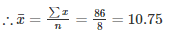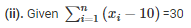(x1−10)+(x2−10)+ ………… + (xn−10) = 30

⇒(x1+x2+x3+x4+x5+⋅⋅⋅+xn)−(10+10+10+10+⋅⋅⋅⋅+10) = 30

⇒ ∑x−10n = 30⋅⋅⋅⋅⋅⋅⋅⋅⋅⋅⋅⋅⋅⋅⋅(1)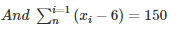⇒(x1−6)+(x2−6)+⋅⋅⋅⋅⋅+(xn−6) = 150

⇒(x1+x2+⋅⋅⋅+xn)−(6+6+6+⋅⋅⋅+6) = 150

⇒∑x−6n = 150⋅⋅⋅⋅⋅⋅⋅⋅⋅(2)

By subtracting equation (1) from equation(2) , we get

∑x−6n−∑x+10n = 150−30

⇒ 4n = 120

⇒ n = 120/4 = 30

Put value of n in equation (1)

∑x−10×30 = 30

⇒ ∑x−300 = 30

⇒ ∑x=30+300 = 330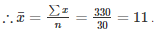Q 22 . The sum of the deviations of a set of n values x1,x2,x3,⋅⋅⋅,xn measured from 15 and -3 are -90 and 54 respectively . Find the value of n and mean .

SOLUTION :

Given :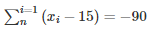⇒ (x1−15)+(x2−15)+⋅⋅⋅⋅⋅+(xn−15) = −90

⇒ (x1+x2+⋅⋅⋅⋅+n)−(15+15+15+⋅⋅⋅⋅⋅⋅+15) =−90

⇒ ∑x−15n =−90⋅⋅⋅⋅⋅(1)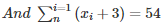⇒ (x1+3)+(x2+3)+⋅⋅⋅⋅⋅+(xn+3) = 54

⇒ (x1+x2+⋅⋅⋅⋅+n)+(3+3+3+⋅⋅⋅⋅⋅⋅+3) = 54

⇒ ∑x+3n = 54⋅⋅⋅⋅⋅(2)

By subtracting equation (1) from equation(2) , we get

∑x+3n−∑x+15n = 54+90

⇒ 18n =144

⇒ n =144/18 = 8

Put value of n in equation(1)

∑x−15×8 = −90

∑x−120 = −90

∑x = 120−90 = 30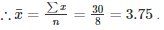Q 23 . Find the sum of the deviations of the variate values 3 , 4 , 6 , 7 , 8 , 14 from their mean.

SOLUTION :

Values 3 , 4 , 6 , 7 , 8 , 14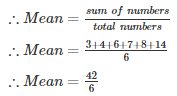= 7

∴ Sum of deviation of values from their mean

= (3−7)+(4−7)+(6−7)+(7−7)+(8−7)+(14−7)

= – 4 – 3 – 1 + 0 + 1 + 7

= – 8 + 8 = 0

Q 24 . If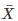is the mean of the ten natural numbers x1,x2,x3,⋅⋅⋅,x10 show that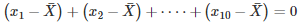SOLUTION :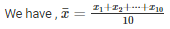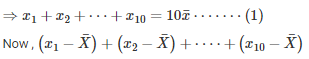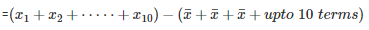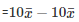[Byequation(i)]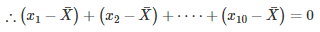Offer running on EduRev: Apply code STAYHOME200 to get INR 200 off on our premium plan EduRev Infinity!

91 docs

,

,

,

,

,

,

,

,

,

,

,

,

,

,

,

,

,

,

,

,

,

,

,

,

,

,

,

,

,

,

,

,

,

;